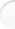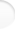Structure of Magic and Semi-Magic Squares, Methods and Tools for Enumeration by Francis GaspalouIn this page, the main results of my paper are presented, without (or with very little) explanations. These explanations are detailed in specific developments further in the text. Most of these results seem original.

It is well known for mathematicians that magic squares have a vector space structure. But it is less well known that magic squares have also a G-set structure (a group G operates on the set of magic squares; transformations on magic squares have a group structure).

The order of group G is the number of variations of a given square. Then enumeration can be reduced to a set of "elementary squares" or "essentially different squares".

For semi-magic squares of order n, the order of group G is 2 (n!)2.
For magic squares of order n=2p or n=2p+1, the order of groups G is 2p+2 (p!) and these two groups are isomorphic; they have the same abstract definition. The first values are:

Order
of squares
Order
of group G
38
432
532
6192
7192
81 536

Some results on group G ( note: I don't divide by 8 the number of squares, as it is usually done in the literature)

SetTotal number
of squares
Order
of group G
Number
of essentially
different squares
Total number
of isomorphic sets
(with initial
set included)
Magic squares of order 47 040322201
Magic squares of order 52 202 441 7923268 826 3061

Semi-magic squares of order 4549 5041 1524771

Semi-pandiagonal squares of order 43 4561 15231
Pandiagonal squares of order 4384384111(1)
Symmetrical squares of order 4384384111(1)
...............

Symmetrical squares of order 5388 3521283 0343
Pandiagonal squares of order 528 80028 80012
de la LOUBERE's squares of order 55 7605 76018
BACHET's squares of order 51 1521 15214
...............

Symmetrical pandiagonal
squares of order 7
161 525 472961 682 557?

(1) there are 9 semi-pandiagonal + 2 other sets

I found the exact mathematical definition of some groups G, as:
• the group G of order 32 for magic squares of order 4 is G 32 n° 7 of BURNS and has for "abstract definition":
s14 = s24 = s32 = s42 = s13 s2 s1 s2 = s3 s1 s3 s13 = s23 s1 s2 s1
= (s4 s1)2 = (s4 s2)2 = (s4 s3)2 = (s3 s2)2 = 1.
• the group G of order 1 152 for semi-pandiagonal squares of order 4 is the only group of order 1 152 of degree 8 and has for "abstract definition":
s112 = s22 = (s14 s2)2(s18 s2) 2 = (s13 s2)2(s19 s2) 2 = (s13 s2 s14 s2) 2 = 1
and I identified examples of generators.

A same set of squares has a group G (transformations on the position of cells) and a group G' (permutations on the contents of cells). E.g.:

SetOrder of group G
(transformations)
Order of group G'
(permutations)
7 040 magic squares of order 4322
3 456 semi-pandiagonal of order 41 152384
384 pandiagonal squares of order 4384384

If the set has a group structure, there is probably equality between the order of group G and the order of group G' (and the dimension of the set).

The transformation and permutation methods can be combined to enumerate the squares in a given set.

E.g.: if you apply the transformation method on the 7 040 magic squares of order 4, you find 220 elementary squares. But these 220 elementary squares can be reduced to 166 if you apply afterwards the permutation method. 166 is the minimum number of elementary squares you can have.

The order of group G is naturally less than the dimension of the set of squares. There is equality for pandiagonal squares of order 4 and 5, but not for superior orders:

OrderNumber
of pandiagonal squares
Order of group GOrder of the subgroup
of transpositions
4384384128
528 80028 800800
60irrelevantirrelevant
7? (estimated by Walter TRUMP)2 352?2 352

The intermediate square method which consists to decompose a square into two squares (capital letters and lower-case letters) is very fruitful to represent some sets of squares, as regular and semi-regular squares.

If a Greco-Latin square of order n can be built, then it is possible to associate k (n!)² semi-magic squares to it, k being the number of orderly basis. This number k is a function of n:

nk
32
46
52
614
72
820

The k (n!)² squares in the associated set are not only semi-magic. Their real properties depend on those of the original Greco-Latin square. For example:
• the 3 456 semi-pandiagonal squares of order 4 are generated from one Greco-Latin square which is semi-pandiagonal,
• the 28 800 pandiagonal squares of order 5 are generated from one Greco-Latin square which is pandiagonal,
• ...

The 2 (n!)² squares given by a Greco-Latin square of order n (with one basis and its corresponding basis) have probably a group structure.

I calculated the first sets of regular squares (with all the basis):

Order 4Order 5Order 6Order 7
Number of regular squares
(with all the basis)

3 456 (semi-pandiagonal)

57 600
(pandiagonal and one other isomorphic set)
0

2 438 553 600
(pandiagonal and 7 other isomorphic sets)

(For order 7, I am not absolutely sure that pandiagonal squares with their isomorphic sets are the only regular squares).

For magic squares of order 6, I found orthogonal squares to Latin diagonal lower-case squares. I calculated the total number of such squares (for all the basis), as well as the equivalent numbers for orders 4 and 5:

Order 4Order 5Order 6
Number of orthogonal squares
to Latin diagonal squares
3 456
(semi-pandiagonal)
410 880

56 005 447 680

Among these orthogonal squares of order 6, I found an intermediate square that generates 483 840 squares, which is for the moment the largest set of squares that can be generated by an intermediate square of order 6.

For magic squares of order 6 too, I found squares with 8 regular lines (6 rows and 2 diagonals for example), which seems to be the maximum possible number.

For symmetrical pandiagonal squares of order 7, I found a new transformation W of order 2 allowing to define a group G of order 96 (and then to reduce by 2 the computation time for enumeration).

For symmetrical pandiagonal squares of order 7, I found squares with 24 irregular lines, which is the maximum possible number.

All the magic squares and semi-magic squares can be generated from elementary capital and lower-case letter squares. I drove such a task for order 4 (for superior orders, it is naturally impossible to do it up to the very end). For example, I found that all the 7 040 squares of order 4 can be generated from 22 elementary squares (with the first basis).

I showed that magic squares of order 4 and semi-magic of order 4 can be decomposed in basic sets, each set being a part of another set before, with a graph of filiation and with isomorphic sets. I think - and it is only a conjecture - it is true also for order n.